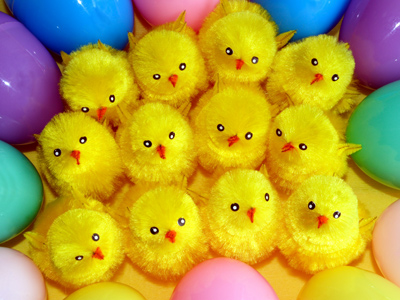The number 12 can be divided by 1, 2, 3, 4 and 6 exactly.

# Multiples (Year 4)

As children progress through KS2 they will learn more about multiples in Maths. As well as being familiar with the multiples of the numbers 2, 5, 10, 50 and 100 , in Year Four children will be exposed to the multiples of other numbers corresponding to their increasing knowledge of times tables. They will also come to learn how to work out multiples using division as well as multiplication.

A multiple is a number resulting from the multiplication of two other numbers. You can find which numbers a multiple is of by using division. If a number can be divided by another number with no remainder then it is a multiple of that number. For example, 25 and 30 are multiples of 5 because they both can be divided by 5. Did you know that the number 0 is a multiple of everything?

Take this quiz for 8-9 year olds and test your knowledge of multiples found by multiplication and division.

1.
Which of these numbers is a multiple of 5?
21
28
30
36
All multiples of 5 end in 0 or 5
2.
Which multiple of 4 comes after 32?
36
38
40
44
3.
Which number can be divided by 2 and 3 exactly?
5
8
9
12
2 x 3 = 6 so all multiples of 6 are also multiples of 2 and 3
4.
All multiples of 10 end in which number?
0
1
2
5
The multiples of 10 are 10, 20, 30, 40 etc
5.
Which number is divisible by 3?
10
13
18
22
All numbers that are divisible by 3 are multiples of 3
6.
Which number is divisible by 4 and 5?
15
16
20
25
4 x 5 = 20
7.
Which number can be divided by 2 exactly?
215
267
289
292
All multiples of 2 are even numbers
8.
Which of these numbers is a multiple of 4?
13
15
16
18
16 ÷ 4 = 4
9.
Which is the next number after 15 that is a multiple of 5 and 3?
18
20
30
45
15 is the lowest multiple of 3 and 5 so the next lowest will be 2 x 15 which is 30
10.
Which multiple of 3 comes before 21?
12
15
18
20
21 - 3 = 18